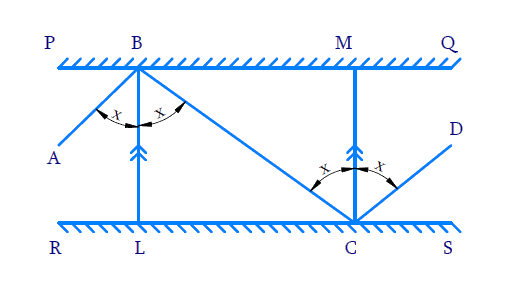# Ex.6.2 Q6 Lines and Angles Solution - NCERT Maths Class 9

Go back to  'Ex.6.2'

## Question

In the given figure, $$PQ$$ and $$RS$$ are two mirrors placed parallel to each other. An incident ray $$AB$$ strikes the mirror $$PQ$$ at $$B$$, the reflected ray moves along the path $$BC$$ and strikes the mirror $$RS$$ at $$C$$ and again reflects back along $$CD$$.

Prove that $$AB \|CD$$.Video Solution
Lines And Angles
Ex 6.2 | Question 6

## Text Solution

What is known?

$$PQ \| RS$$

What is unknown?

To prove: $$AB \| CD$$

Reasoning:

When two parallel lines are cut by a transversal, alternate angles formed are equal.

In optics the angle of incidence (the angle which an incident ray makes with a perpendicular to the surface at the point of incidence) and the angle of reflection (the angle formed by the reflected ray with a perpendicular to the surface at the point of incidence) are equal.

Steps:

Draw perpendicular lines $$BL$$ and $$CM$$ at the point of incident on both mirrors since $$PQ$$ and $$RS$$ parallel to each other, perpendiculars drawn are parallel $$BL \| CM$$. Since $$BC$$ is a transversal to lines $$BL$$ and $$CM$$, alternate angles are equal so we get

$\angle LBC = \angle BCM = x \;(\text{say})\; \ldots ( 1 )$

By laws of reflection, at the first point of incidence $$B$$, we get:

\begin{align} \angle ABL & = \angle LBC = x \\ \therefore \angle ABC& = \angle ABL+ \angle LBC \\ & = x + x \\ \therefore \angle ABC & = 2 x \ldots \ldots \ldots ( 2 ) \end{align}

By laws of reflection, at the first point of incidence $$C$$, we get:

\begin{align} \angle MCD & = \angle BCM = x \\ \therefore \angle BCD& = \angle BCM + \angle MCD \\ & = x + x \\ \angle BCD &= 2 x \ldots \ldots ( 3 ) \end{align}

From equations ($$2$$) and ($$3$$), we get $$\angle ABC = \angle BCD$$.

We know that, if a transversal intersects two lines such that a pair of alternate interior angles is equal, then the two lines are parallel. As Alternate angles are equal we can say $$AB \| CD$$.

Video Solution
Lines And Angles
Ex 6.2 | Question 6

Learn from the best math teachers and top your exams

• Live one on one classroom and doubt clearing
• Practice worksheets in and after class for conceptual clarity
• Personalized curriculum to keep up with school